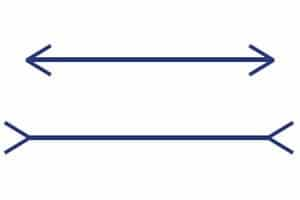# Framing

Framing refers to the observation that people’s decisions tend to be affected by the way in which the choices are framed. One important case in which framing occurs is the following. Individuals may behave risk averse in terms of potential gains but behave risk seeking in terms of potential losses.

Let’s consider two examples to illustrate this behavioral bias. The first one illustrates the concept of framing visually, the second example provides an example that explains the concept in a financial context.

## Visual framing example

To get the intuition behind framing, consider the figure below. Do you think the lines have the same length? While both lines appear to be different in length, this is actually not the case. You can measure the length of both lines. This is an optical illusion, but illustrates the concept of framing in an intuitive way.## Finance framing example

Next, we consider a financial example. Suppose we create following experiment. To some of the participants, we propose the following coin toss. In the case of tails, the payoff is 50, and zero otherwise. Alternatively, we give some participants a gift of 50, but they also have to participate in a coin toss that imposes a loss of 50 when the coin toss comes up heads.

The way the above experiment is structured, you end up with zero in the case of heads, and 50 in the case of tails. As such, the payoff is the same in both cases. Still, the first description ‘frames’ the coin toss as a risky gain, while the second situation ‘frames’ the coin toss as a risky loss. The consequence will be that most people will agree to the first gamble, but not to the second.

## Summary

We have discussed framing, a behavioral bias that causes people to accept or decline proposals depending on the the way they are framed. Even though the payoff is the same, people will agree to proposals framed as risky gains, but will decline the proposals described as risky losses.